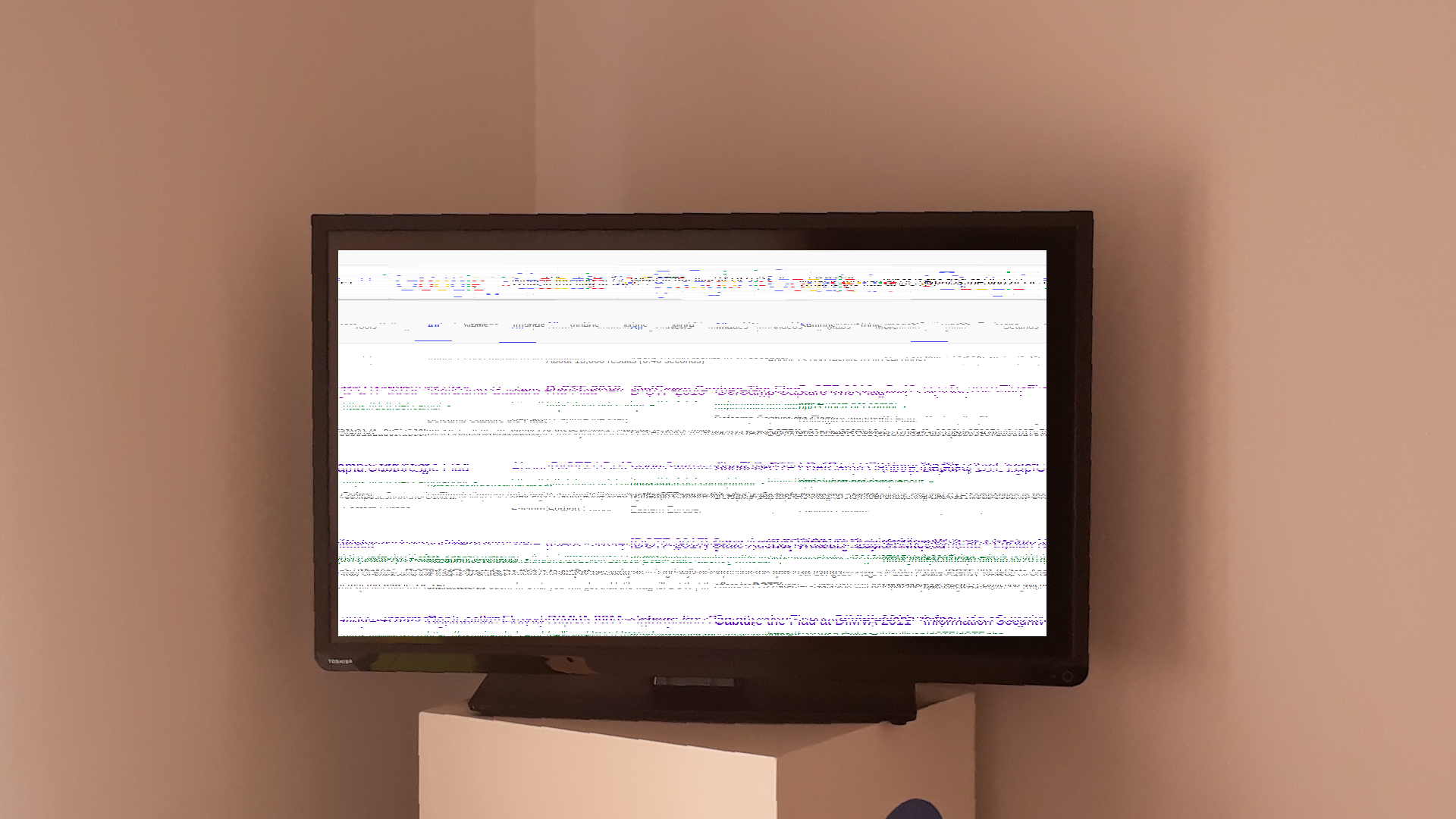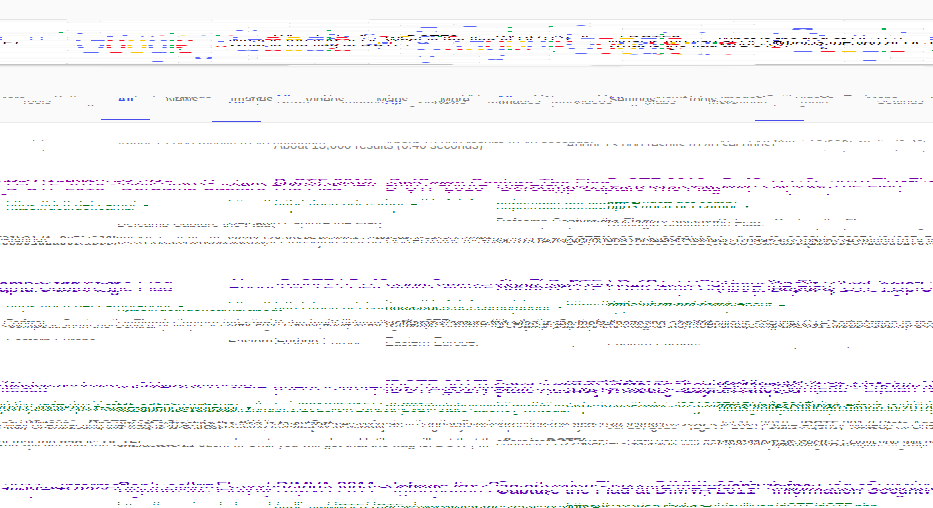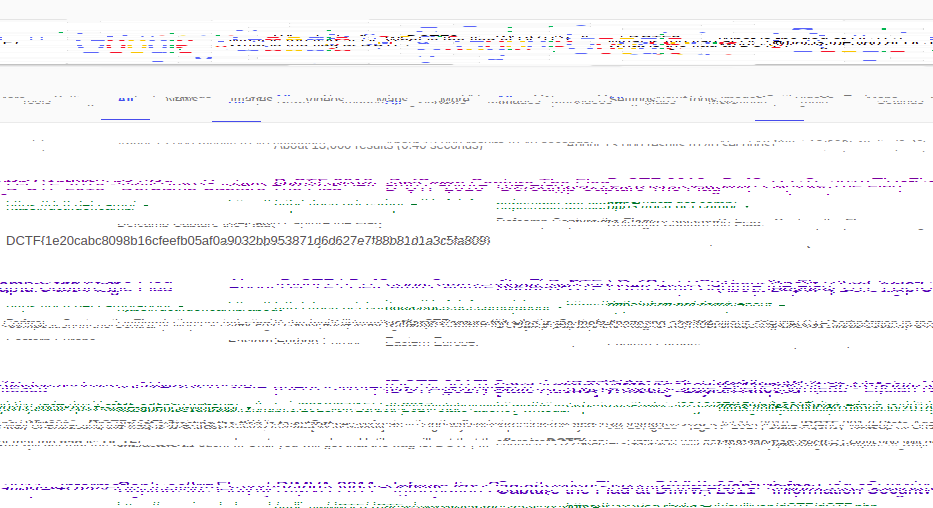# pwnthem0le

## pwnthem0le is a Turin-based, hacking students group born out of CyberChallenge 2018. Read more about us!

28 September 2018

# DefCamp CTF Qualification 2018 - Broken TV Writeup

by matpro98

The challenge gives us this image:Watching in detail the pixels, we can convince ourselves that the image is simply shifted by rows.

We start by isolating the display (in Python):

from PIL import Image

im=Image.open('dctfbrokentv_1.png')
im=im.crop((446,330,1379,838))
im.save('dctfbrokentv_2.png')


The code returns this image:Now, let’s start guessing the rows to shift and how much we have to shift them. After a few attempts, we find the flag:The code is the following:

from PIL import Image

def roll(image, delta,y):
xsize, ysize = image.size
ysize=y
delta = delta % xsize
if delta == 0: return image
part1 = image.crop((0, ysize, delta, ysize+1))
part2 = image.crop((delta, ysize, xsize, ysize+1))
image.paste(part2, (0, ysize, xsize-delta, ysize+1))
image.paste(part1, (xsize-delta, ysize, xsize, ysize+1))
return image

shift=
for i in range(506):
shift.append(0)

shift=560
shift=222
shift=490
shift=379
shift=490
shift=111
shift=712
shift=560

im=Image.open('dctfbrokentv_2.png')
for i in range(507):
roll(im,shift[i],i)

im=im.convert('RGB')
im.save('dctfbrokentv_3.png')


DCTF{1e20cabc8098b16cfeefb05af0a9032bb953871d6d627e7f88b81d1a3c5fa809}

tags: steganography  D-CTF  misc